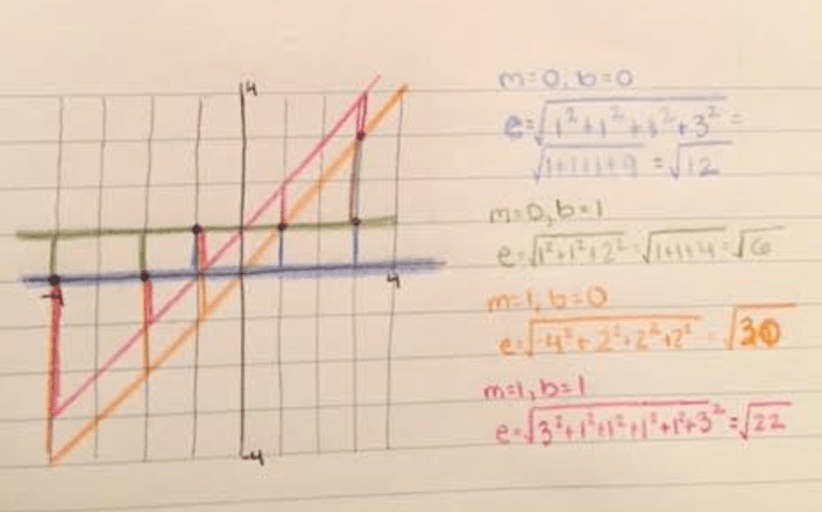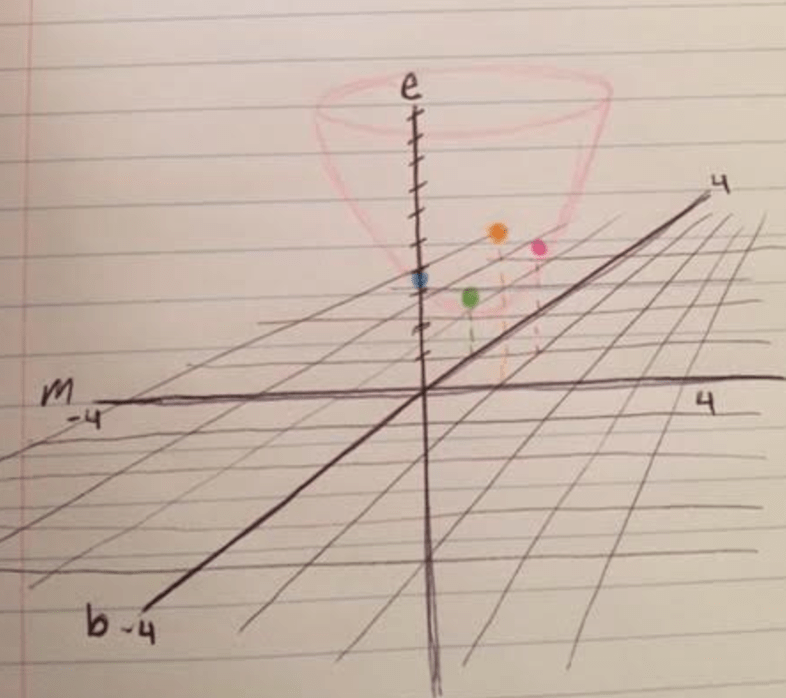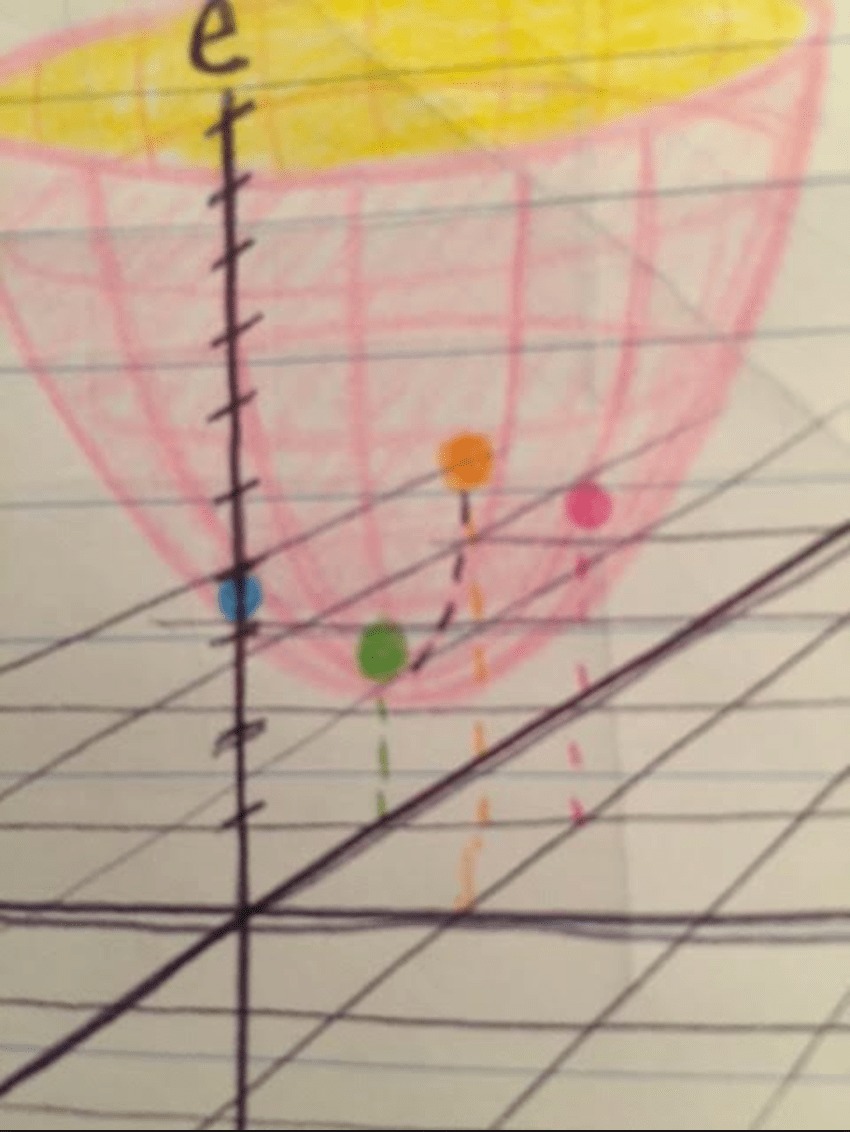# Machine Learning Intuition: Cost Function Optimization

Suppose you want to identify and describe the patterns that arise in some data.

How do you look for patterns? How do you measure how closely your descriptions match the data? How can you improve your descriptions  of this data? How do you know your description is general enough to describe other data like this data?

Those are the questions that machine learning tasks venture to answer. They sound less sexy than the headlines in WIRED, but we can use machine learning to solve tough quantitative problems in a wide range of fields, from insurance to travel to medicine.

There are a plethora of approaches and algorithms in machine learning. You can approach the majority of them once you understand one foundational piece: cost optimization.

Words. What do they mean?

cost – the amount of wrongness in our description of the data.

optimization – reducing the wrongness as much as possible.*

*Ehhh…this is a simplification of the truth. The whole truth involves some more words (bias and variance, if you’re curious). Let’s just stick with the simplification for now and come back to this later.

So for now, we want to reduce the wrongness in our description of the data.

We do that using some math, but I’ll try to illustrate the concept with no advanced math whatsoever (that is, no calculus, no Taylor series, no stepping formula. We still have to do a little algebra and arithmetic).

I have some data like this:And I want to come up with an equation that describes what this data is doing.

So I take a first crack at this by drawing a line like this:How accurately does this line match the data? It’s quite a crude representation, really. There’s a lot of wrongness in this representation. Let’s calculate just how much wrongness there is.

We add up the distance between each of our data points and the line we tried to use to describe them. The sum of these distances gives us a way to quantify the wrongness in our representation.We can also refer to all these little wrongnesses as the error. We square the errors before we add them up. Why? We do this so that below-the-line wrongness does not cancel out above-the-line wrongness in our sum of all the wrongness.  We want positive and negative errors to count toward our sum of errors, and the squares of each wrongness cancel out which direction the wrongness is in, leaving only a measure of how big it is.

So we have a line. The equation for a line is y = mx + b. For any line that we could draw through this data with a slope m and a y-intercept b, we can also calculate a total wrongness, or total error, between that line and the data .

Remember, we can also refer to this total wrongness as the cost of our description. It’s the amount of accuracy we give up in our effort to describe the data this way.

What if we tried a different line?We could be here all day arbitrarily guessing lines and calculating their costs. Luckily, we have a better way of figuring out which line will describe this data most accurately. Enter our cost function.

Let’s make a plot. It’s going to be a 3-D plot, and it will plot all the different lines we’ve tried so far. The x-axis will have slope values, the y-axis will have y-intercept values, and the z-axis will show our total cost.Let’s plot some of the points we found while we were guessing lines. We need a point at (0,0,√12) to represent the error in our blue line, whose slope and y-intercept were both zero. We need one at (0,1,√6) for the error in our green line, whose slope was 0 and y-intercept was 1.

Some points are lower than others on our z-axis, which represents error. Ultimately, to minimize our cost, we need to find the point with the lowest z value. Luckily, there’s a pattern that emerges in our points…If we were to try lots of lines, and plot more points on our 3d graph, the points would all lie on a cost function for describing our collection of data. What do you think that cost function would look like? Would it have a shape?

This little animation can help you look into the future and see what our cost function would look like if we kept plotting points:

(Many thanks to Jeremy Watt for this helpful animation!)

Our cost function in this case looks like a paraboloid. And that paraboloid has a low point on it.Out of the four that we’ve plotted so far, it looks like (m=0,b=1) has the lowest cost. But there might be another point right around there that’s even lower (a local minimum), or somewhere else entirely on the function there could be an even lower dip (a global minimum). So how do we find our lowest point?

We could try to plot every single point on the cost function and look for the lowest point anywhere. This would take a really long time, so instead we’re going to try gradient descent.

Take another look at our paraboloid. Where is the lowest point on it? At that lowest point, what is the function doing? Is the wall of the function steep, or is it flat?

It’s flat! The slope of the cost function is zero at its lowest point because the function is flat there—then the function gets steeper and steeper as it goes up and away from that lowest point.

This is very important. When we do gradient descent, we are looking for flatness in our cost function.

When we do gradient descent, we pick an m value and b value on our cost function, and we figure out how steep the cost function is around that area. Then we travel down the steepness, pick a new point with a lower cost, and do the same thing again. We want to travel down our cost function, step by step, like a droplet of water rolling down the side of a cup until it reaches the bottom. If we started at the orange dot, we would want to descend like this:So how do we do it?

Suppose we start at the orange dot: (1,0,√30). So, let’s try another point near there: say (1/2, 0). When we plot a line with slope 1/2 and y-intercept 0, our error is √10 (about 3.16). That’s an improvement from √30, which is about 5.47. So we’re moving in the right direction on the cost function! Let’s try moving that direction again.

But here’s the thing: if we just reduce m by 1/2 again, we get to (m=0,b=0). And we know that the cost there was √12, which is higher than √10. This is an example of what can happen if we take steps that are too big…we could end up bouncing around our cost function and not finding the bottom of it. So instead let’s take a smaller step: let’s try (m=1/4,b=0). When we draw that line and count up all of our errors, we find the cost there to be about √7.5. Even smaller error! Let’s try lowering the slope again and calculate the error at (m=1/5,b=0). Our error here is…√8.8. It’s larger than at m=1/4. So, let’s try increasing the slope a little instead. We don’t want to increase all the way to 1/2, because that gave us a larger error of √10. So let’s pick something in between…say, m=0.3. Here, we get an even lower error, though not by very much. When the change in the amount of error gets smaller for each step we take, that’s an indication that the cost function is flattening out…and that we’re approaching a minimum. Sometimes researchers will take smaller steps as the differences in cost get smaller with each step, to make sure they don’t overshoot the minimum by accident. Also, sometimes researchers will call it close enough if the cost isn’t changing much anymore as they move around.

For our cost function, let’s stick with m=0.3 as the slope for now. The whole time we were trying different slopes, we kept the y-intercept constant at 0. We can do the same exercise with the y-intercept, holding the slope constant at 0.3 and trying out y-intercepts until our error is as low as we’ve seen and isn’t changing much when we try y-intercept values that are close by. Try finding the y-intercept for the best fit line to this data on your own. What do you find?

You might know that there’s a formula we can use to find the best fit straight line given some data points. So why did we use gradient descent? Well, a best-fit straight line has relatively few variables in it, so we can visualize what’s going on as we learn the concept of cost optimization. Unlike the best-fit straight line formula, though, gradient descent can be used for cost optimization in many different cases. We could use it to fit a polynomial or logistic regression curve to some data, for example.

There is one other thing about gradient descent. If we’re looking for low points on our function, couldn’t gradient descent give us the wrong model if the cost function looked like this?We might find that local minimum and have no idea there’s an even lower point somewhere else. In practice, machine learning researchers will try to avoid this by starting gradient descent at several different points and fitting the model using the lowest error they find from all of their gradient descents.

Also in practice, though, the cost functions for many machine learning models are concave up—which means that they curve upward and have just one minimum across their entire range. They look like the pink and yellow paraboloid we drew up above. So, while researchers take precautions against getting stuck in local minima with gradient descent, they can also identify when they’re not likely to run into that problem.

In practice, machine learning practitioners will use an algorithm to do gradient descent. They’ll pick a point, find the derivative of the cost function at that point (which describes the cost function’s slope), and travel in the downward direction, pick a new point, and repeat. They’re using the derivative to decide which direction to go, because the slope tells them which way the function is going down. This is what we did when we found the error at (m=1,y=0), then tried a point with a lower m value of 1/2, found that our error was lower in that direction, and tried lowering the m value again. This is also what we did when we tried lowering the m value from 1/4 to 1/5, realized our error was going up, and decided to step in the other direction (to m=0.3) instead.

Machine learning practitioners also sometimes use the derivative to decide how far to go; if the function is really steep, then we’re not so close to a minimum and so we can take bigger steps to get there faster. If the function is not so steep, then we know we’re close and we take smaller steps so we don’t overshoot it. This is what we did when we realized that error went down when we moved from m=1 to m=1/2, but we noticed that if we went down by 1/2 again the error would go up. So instead, we lowered the error by a smaller amount. Our steps got shorter as we got closer to the minimum. This is common when performing gradient descent.

There are a number of fancy ways to optimize gradient descent, but you have now done your own gradient descent by hand: you guessed a point, gathered a little bit of information, and used that information to guess your next point. This general cost optimization strategy shows up throughout machine learning, so when you fit models with machine learning libraries in the future, you’ll have an idea of how those models get fitted under the hood.

1.Sahil Maheshwari says:
2.Hannan says: